# Correlation

### General

A correlation is the degree of similarity between a signal and itself (Auto Correlation) or between 2 different signals (Corss Correlation). A signal is a set of values over time. So if a Analog Digital Converter (ADC) is giving us a value of e.g. a voltage in a determined interval, this set of values is called a signal. Those values are also called samples as they are a sample out of the continuous time domain function. The inverse of this so called sampling period  is the sampling frequency.

Correlation is used in a lot of applications, whenever there is the need to check if a signal is periodic or if we want to check if a known signal is hidden within another signal.

#### continuous Time domain

The ACF is used to correlate a periodic signal with itself. A periodic signal will always create a periodic output. Imagine you have a periodic signal embedded in noise and you want to extract it.
The general definition of the ACF in the time domain is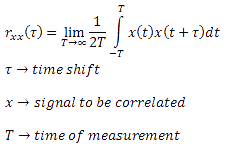you can see that the index is xx so you see that its the correlation of the signal x with signal x

first we want to demonstrate that it is possible to get our signal p(t) out of a noisy environment n(t)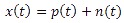so lets analyze whats in there: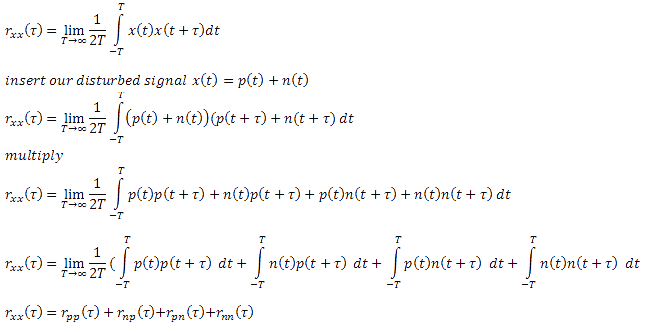You see that we get 4 correlations which are inside.
2 crosscorrelations (np and pn). Those signals are completely uncorrelated and therefore 0
2 autocorrelations (nn and pp)
here things get a bit more interesting. nn is uncorrelated (0) or at least it should show a very low correlation). At tau=0 the correlation of course is having its maximum as for every ACF.
pp is the signal we were searching for. It will show a high correlation and swap the low correlation of nn.

#### discrete Time domain

Of course we can not apply this to our digital world as we have to handle with discrete values which will form the signal. An Integral in the digital world is a summation so we get: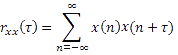Please see the slides too see how the signals are look alike.
If a signal is buried in noise you could do a FFT to get the frequency components. You reach a better S/N ratio if you first do a ACF and then a FFT of it.

[size=150]Cross Correlation Function CCF[/size] At CCF 2 signals will be correlated. As for the ACF the single values of the signal are multiplied by one another and then shifted for values of tau.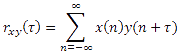Example: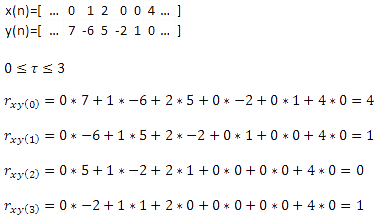#### Application

Lets say we have a Radar System where we have to get the distance between our transceiver and an object. We know that we will have an time delay between the send and received signal which is equal to 2 times the distance (there and back)divided by the speed of light.

we do send a signal s(t) which will be reflected by an object and come back delayed s(t-T) also the amplitude had change so it is as(t-T). Also there is noise added to the signal. We can say that our received signal r(t)=as(t-T)+n(t)

Lets try to get T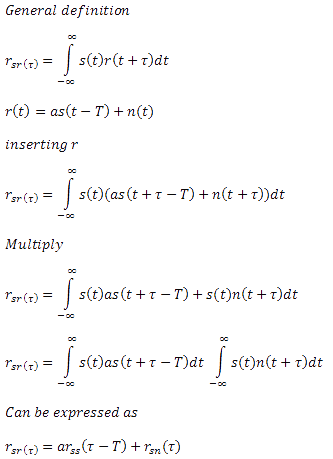as s and n are uncorrelated this will not influence the result because its 0.
From the autocorrelation of s we will get the shift between the orginal and the delayed signal which represents T and with the knowledge of c we know can calculate the distance R.

This site uses cookies. By continuing to browse the site you are agreeing to our use of cookies.